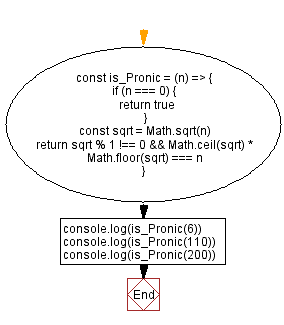# JavaScript: Pronic number

## JavaScript Math: Exercise-72 with Solution

Write a JavaScript program to check if a given number is pronic using the product of two consecutive numbers. If the number is pronic return true otherwise false.

From Wikipedia –
A pronic number is a number that is the product of two consecutive integers, that is, a number of the form n(n+1). The study of these numbers dates back to Aristotle. They are also called oblong numbers, heteromecic numbers, or rectangular numbers; however, the term "rectangular number" has also been applied to the composite numbers.
The first few pronic numbers are:
0, 2, 6, 12, 20, 30, 42, 56, 72, 90, 110, 132, 156, 182, 210, 240, 272, 306, 342, 380, 420, 462 ...

Sample Data:
6 -> true
110 -> true
200 -> false

Sample Solution:

HTML Code:

``````<!DOCTYPE html>
<html>
<meta charset="utf-8">
<title>JavaScript Pronic number</title>
<body>

</body>
</html>
```
```

JavaScript Code:

``````const is_Pronic = (n) => {
if (n === 0) {
return true
}
const sqrt = Math.sqrt(n)
return sqrt % 1 !== 0 && Math.ceil(sqrt) * Math.floor(sqrt) === n
}

console.log(is_Pronic(6))
console.log(is_Pronic(110))
console.log(is_Pronic(200))
```
```

Sample Output:

```true
true
false
```

Flowchart:Live Demo:

See the Pen javascript-math-exercise-72 by w3resource (@w3resource) on CodePen.

Improve this sample solution and post your code through Disqus

What is the difficulty level of this exercise?

Test your Programming skills with w3resource's quiz.

﻿